2015-08-22 16:13:12 Kena_M 阅读数 3908
• ###### 机器学习之概率与统计推断

本课程讲解机器学习算法所需概率和统计推断知识。概率部分包括概率公理及推论、条件概率、贝叶斯公式、随机变量及其概率函数（CDF／pdf）、常用概率分布及其均值、方差；统计推断部分包括大数定律和中心极限定理、极大似然估计、贝叶斯估计，估计的评价、偏差-方差平衡。课程还会讲解假设检验的基本概念。

20464 人正在学习 去看看 AI100讲师

​忙里偷闲，整理了一下之前看的材料和自己实现的代码，这次说说均值滤波的事儿。

## 来看一个快速的均值滤波算法思路：

average=sumN×M

sum=sumj=M/2M/2g(xN2,y+j)+j=M/2M/2g(x+1+N2,y+j)

j=M/2M/2g(xN2,y+j)$\sum\limits_{j=-M/2}^{M/2} g(x-\frac{N}{2},y+j)$是去掉的左边列的像素的累加和；
j=M/2M/2g(x+1+N2,y+j)$\sum\limits_{j=-M/2}^{M/2} g(x+1+\frac{N}{2},y+j)$是新添的右边列的象素的累加和；

##### 好了，有这些就够了，下面上代码

/**
* @brief Fast mean filter
* @param src
* @param width
* @param height
*/
void meanBlur(unsigned char* src, int width, int height, int maskw, int maskh)
{
int i, j, l, temp;
int width1 = width + maskw - 1;
int height1 = height + maskh - 1;
int *sumCol = NULL;
int *psum, *ps;
unsigned char *pCurOrg, *pCurObj, *pu, *pd;
unsigned char *src1 = NULL;
sumCol = Malloc(int, width1);
memset(sumCol, 0, width1 * sizeof(int));
src1 = Malloc(unsigned char, width1 * height1);
//获得带边界扩展的图像副本
//清空原图像数据
memset(src, 0, width * height * sizeof(unsigned char));
//构造首个横向滑窗列
//sumCol[i] = sum(和滑窗等高的每一列像素灰度)
for(i = 0, psum = sumCol; i < width1; i++, psum++)
for(j = 0, pCurOrg = src1 + i; j < maskh; j++, pCurOrg += width1)
*psum += *pCurOrg;
for(i = 0, pCurObj = src; i < height; i++)
{
psum = sumCol;
temp = 0;
pCurObj = src + i * width;
for(j = 1; j < width; j++, psum++)
{
temp = temp - *psum + *(psum + maskw);
}
for(pu = src1 + i * width1, pd = src1 + (i + maskh) * width1, ps = sumCol, l = 0; l < width1; l++, ps++, pd++, pu++)
*ps = *ps + *pd - *pu;
}
free(sumCol);
free(src1);
}

/**
* @brief Generate a boundary-extended image to mask by boundary-replicate;
* @param src
* @param dst
* @param width
* @param height
* @param nwidth
* @param nheight
* @param new_w
* @param new_h
*/
void boundaryExtention(unsigned char* src, unsigned char *dst, int width, int height, int nwidth, int nheight, int new_w, int new_h )
{
int i;
int half_nw, half_nh;
unsigned char *pCurOrg, *pCurObj;
if(nwidth / 2 != (nwidth - 1) / 2)
{
printf("The mask window width is not odd !\n");
return;
}
if(nheight / 2 != (nheight - 1) / 2)
{
printf("The mask window height is not odd !\n");
return;
}
half_nw = nwidth / 2;
half_nh = nheight / 2;
if(new_w != width + nwidth - 1 || new_h != height + nheight - 1)
{
printf("The extended image space caculated is wrong!");
return;
}
memset(dst, 0, new_w * new_h * sizeof(unsigned char));

//水平对拷
for(i = 0, pCurOrg = src, pCurObj = dst + half_nw; i < half_nh; i++)
{
memcpy(pCurObj, pCurOrg, width);
pCurObj = pCurObj + new_w;
}

for(i = 0, pCurOrg = src, pCurObj = dst + half_nh * new_w + half_nw; i < height; i++)
{
memcpy(pCurObj, pCurOrg, width);
pCurOrg = pCurOrg + width;
pCurObj = pCurObj + new_w;
}
for(i = 0, pCurOrg = src + (height - 1) * width, pCurObj = dst + (height + half_nh) * new_w + half_nw; i < half_nh; i++)
{
memcpy(pCurObj, pCurOrg, width);
pCurObj = pCurObj + new_w;
}

//垂直对拷
for(i = 0, pCurOrg = dst + half_nw, pCurObj = dst; i < new_h; i++)
{
memset(pCurObj, *pCurOrg, half_nw);
pCurOrg += new_w;
pCurObj += new_w;
}
for(i = 0, pCurOrg = dst + width + half_nw-1 , pCurObj = dst + width + half_nw; i < new_h; i++)
{
memset(pCurObj, *pCurOrg, half_nw);
pCurOrg += new_w;
pCurObj += new_w;
}
return;
}
/**
* @param vect
* @param length
* @return the sum
*/
int sumVect(int *vect, int length)
{
int result=0;
int *p,*pend;
for(p=vect,pend=vect+length;p<pend;p++)
result += *p;
return result;
}

### 经测试，该均值滤波与OpenCV和的结果都是一致的，对于512*512的lena灰度图，耗时3～4ms，，，OpenCV 开挂用了Intel 的IPP库，高富帅，比不起，，，

2018-09-02 23:27:36 Eastmount 阅读数 19739
• ###### 机器学习之概率与统计推断

本课程讲解机器学习算法所需概率和统计推断知识。概率部分包括概率公理及推论、条件概率、贝叶斯公式、随机变量及其概率函数（CDF／pdf）、常用概率分布及其均值、方差；统计推断部分包括大数定律和中心极限定理、极大似然估计、贝叶斯估计，估计的评价、偏差-方差平衡。课程还会讲解假设检验的基本概念。

20464 人正在学习 去看看 AI100讲师

PS：请求帮忙点个Star，哈哈，第一次使用Github，以后会分享更多代码，一起加油。

1.图像平滑
2.均值滤波
3.方框滤波
4.高斯滤波
5.中值滤波

PS：本文介绍图像平滑，想让大家先看看图像处理的效果，后面还会补充一些基础知识供大家学习。文章参考自己的博客及网易云课堂李大洋老师的讲解，强烈推荐大家学习。

PSS：2019年1~2月作者参加了CSDN2018年博客评选，希望您能投出宝贵的一票。我是59号，Eastmount，杨秀璋。投票地址：https://bss.csdn.net/m/topic/blog_star2018/index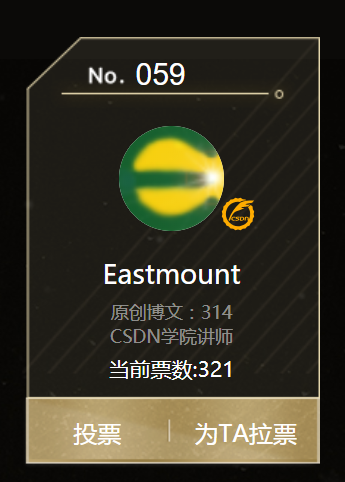# 一.图像平滑

1.图像增强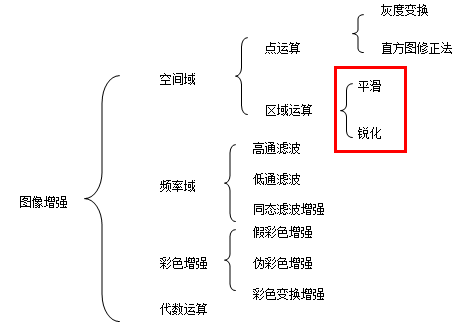2.图像平滑

3.邻域平均法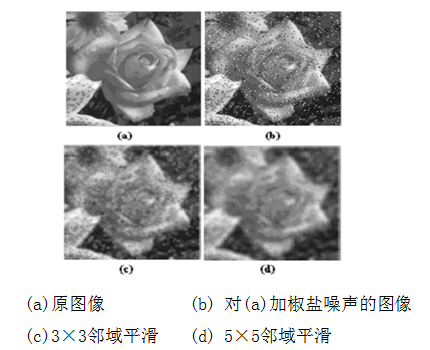# -*- coding:utf-8 -*-
import cv2
import numpy as np

#读取图片
rows, cols, chn = img.shape

#加噪声
for i in range(5000):
x = np.random.randint(0, rows)
y = np.random.randint(0, cols)
img[x,y,:] = 255

cv2.imshow("noise", img)

#等待显示
cv2.waitKey(0)
cv2.destroyAllWindows()# 二.均值滤波

1.原理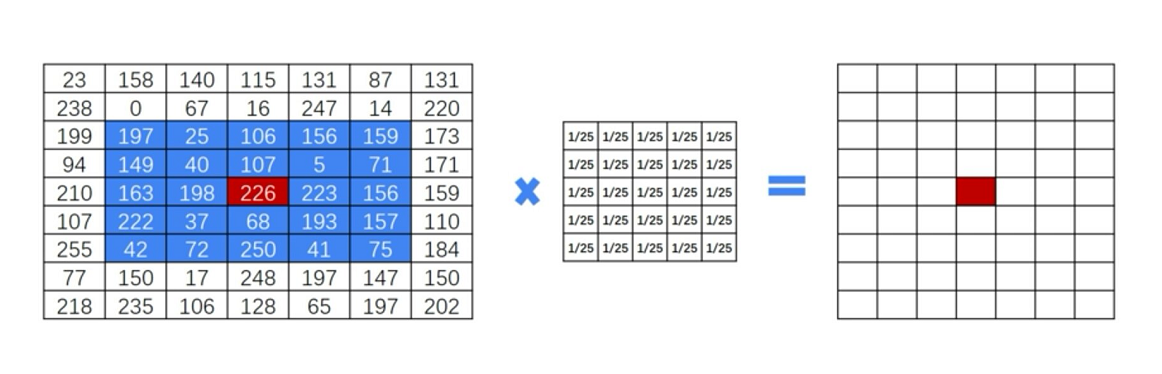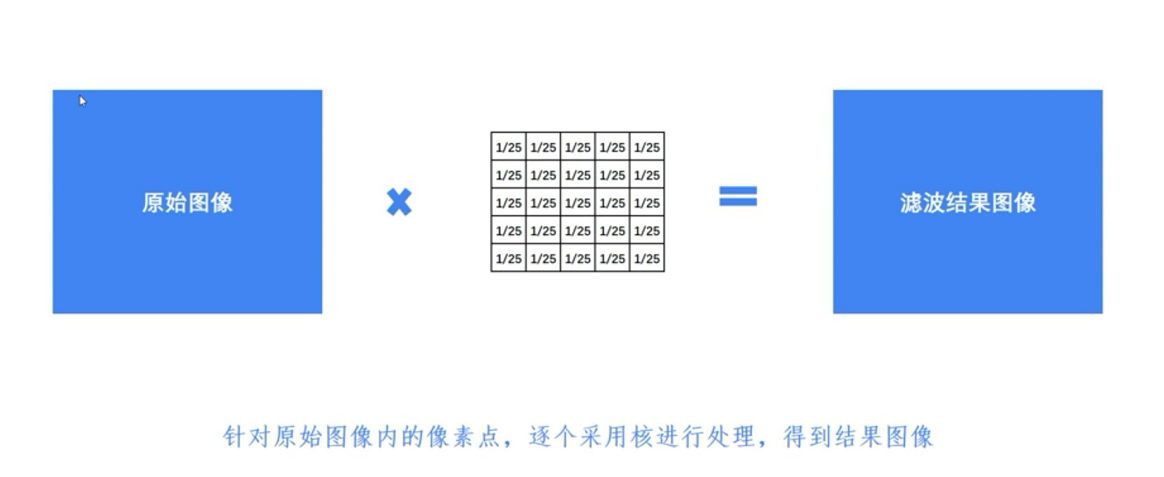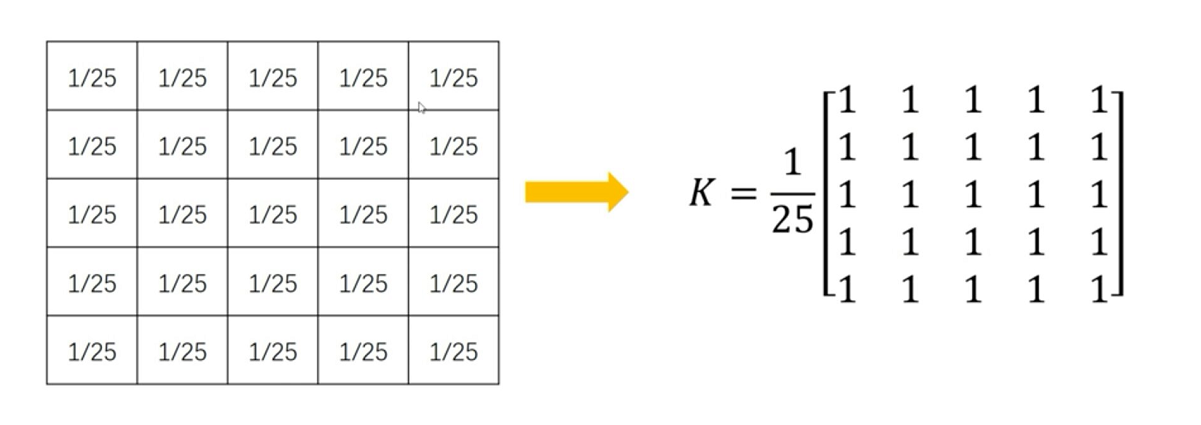2.代码
Python调用OpenCV实现均值滤波的核心函数如下：
result = cv2.blur(原始图像,核大小)

$K=\frac{1}{9}\left[ \begin{matrix} 1 & 1 & 1 \\ 1 & 1 & 1 \\ 1 & 1 & 1 \end{matrix} \right] \tag{1}$
$K=\frac{1}{25}\left[ \begin{matrix} 1 & 1 & 1 & 1 & 1 \\ 1 & 1 & 1 & 1 & 1 \\ 1 & 1 & 1 & 1 & 1 \\ 1 & 1 & 1 & 1 & 1 \\ 1 & 1 & 1 & 1 & 1 \\ \end{matrix} \right] \tag{2}$

#encoding:utf-8
import cv2
import numpy as np
import matplotlib.pyplot as plt

#读取图片
source = cv2.cvtColor(img,cv2.COLOR_BGR2RGB)

#均值滤波
result = cv2.blur(source, (5,5))

#显示图形
titles = ['Source Image', 'Blur Image']
images = [source, result]
for i in xrange(2):
plt.subplot(1,2,i+1), plt.imshow(images[i], 'gray')
plt.title(titles[i])
plt.xticks([]),plt.yticks([])
plt.show()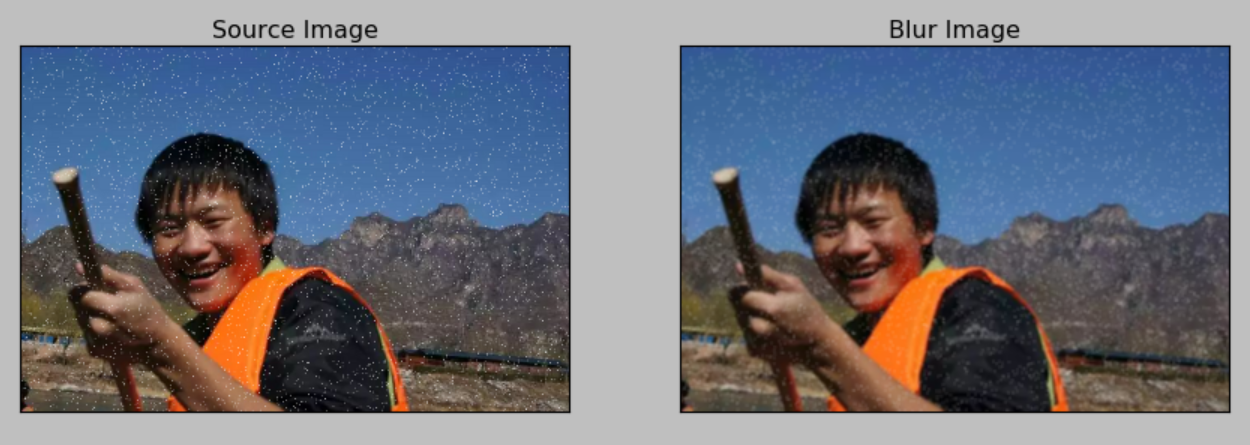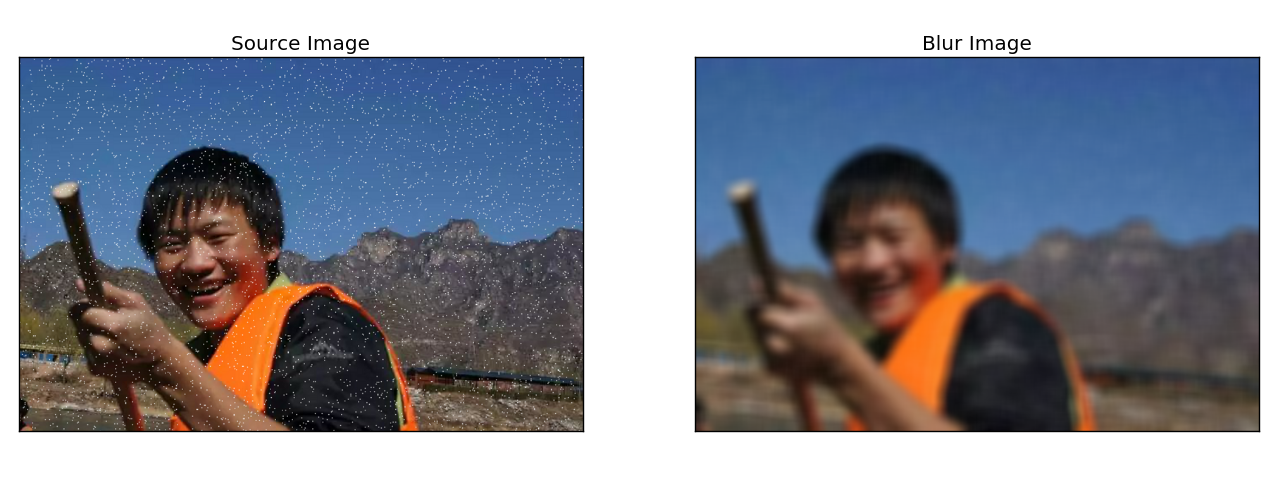# 三.方框滤波

result = cv2.boxFilter(原始图像, 目标图像深度, 核大小, normalize属性)

$K=\frac{1}{9}\left[ \begin{matrix} 1 & 1 & 1 \\ 1 & 1 & 1 \\ 1 & 1 & 1 \end{matrix} \right] \tag{3}$
$K=\frac{1}{25}\left[ \begin{matrix} 1 & 1 & 1 & 1 & 1 \\ 1 & 1 & 1 & 1 & 1 \\ 1 & 1 & 1 & 1 & 1 \\ 1 & 1 & 1 & 1 & 1 \\ 1 & 1 & 1 & 1 & 1 \\ \end{matrix} \right] \tag{4}$

normalize属性表示是否对目标图像进行归一化处理。当normalize为true时需要执行均值化处理，当normalize为false时，不进行均值化处理，实际上为求周围各像素的和，很容易发生溢出，溢出时均为白色，对应像素值为255。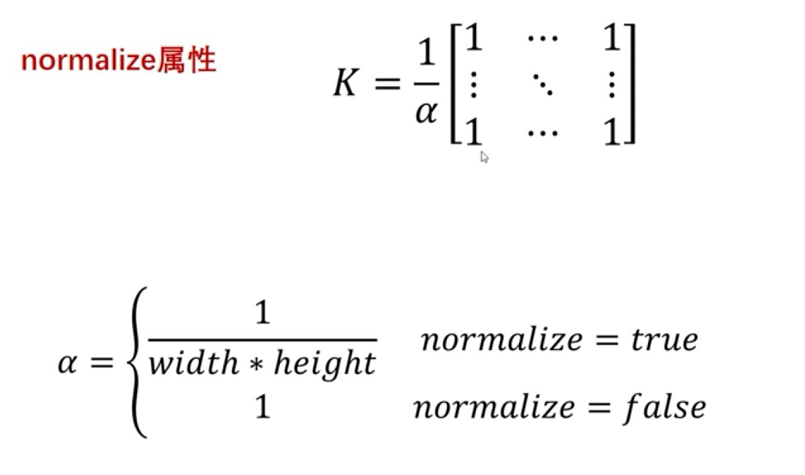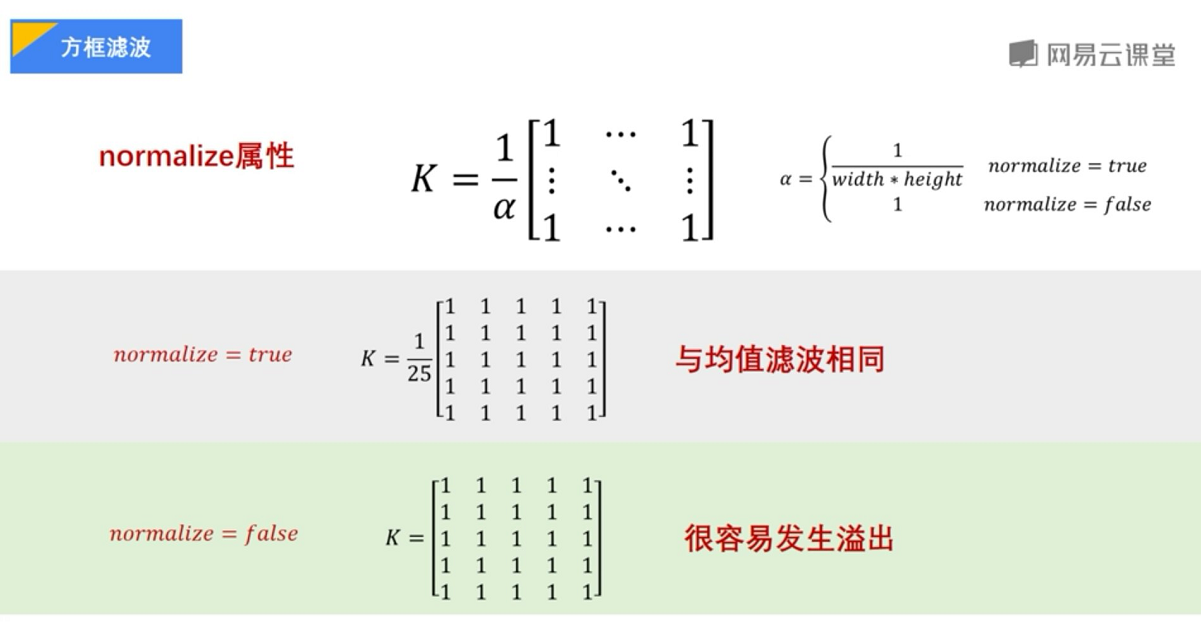#encoding:utf-8
import cv2
import numpy as np
import matplotlib.pyplot as plt

#读取图片
source = cv2.cvtColor(img,cv2.COLOR_BGR2RGB)

#方框滤波
result = cv2.boxFilter(source, -1, (5,5), normalize=1)

#显示图形
titles = ['Source Image', 'BoxFilter Image']
images = [source, result]
for i in xrange(2):
plt.subplot(1,2,i+1), plt.imshow(images[i], 'gray')
plt.title(titles[i])
plt.xticks([]),plt.yticks([])
plt.show()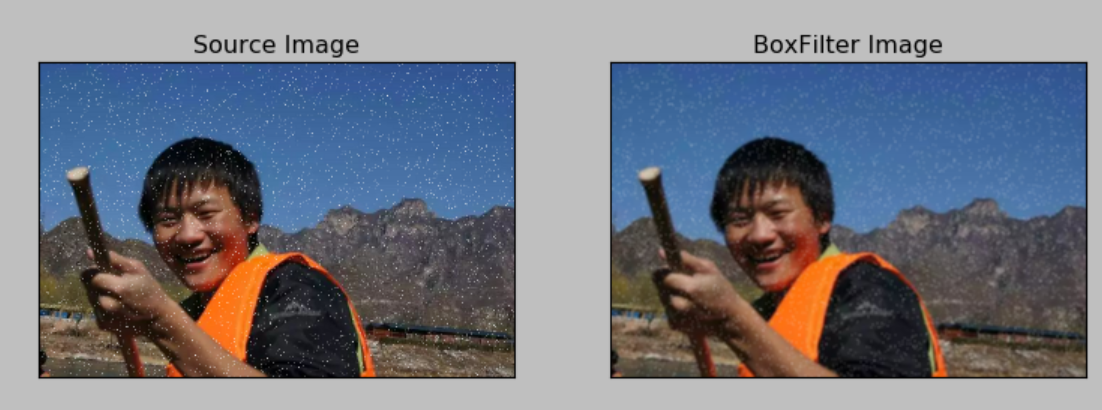print(source[0:3, 0:3, 0])
#[[115 180 106]
# [ 83 152  72]
# [ 55  58  55]]
print(result[0:3, 0:3, 0])
#[[92 90 78]
# [92 89 77]
# [82 80 72]]


#encoding:utf-8
import cv2
import numpy as np
import matplotlib.pyplot as plt

#读取图片
source = cv2.cvtColor(img,cv2.COLOR_BGR2RGB)

#方框滤波
result = cv2.boxFilter(source, -1, (5,5), normalize=0)

#显示图形
titles = ['Source Image', 'BoxFilter Image']
images = [source, result]
for i in xrange(2):
plt.subplot(1,2,i+1), plt.imshow(images[i], 'gray')
plt.title(titles[i])
plt.xticks([]),plt.yticks([])
plt.show()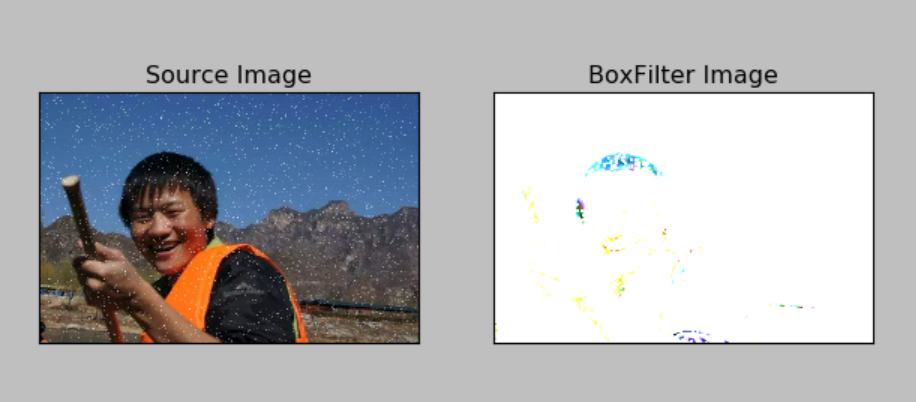result = cv2.boxFilter(source, -1, (2,2), normalize=0)# 四.高斯滤波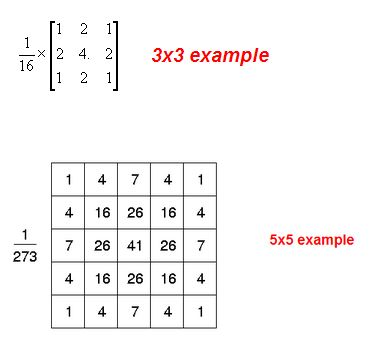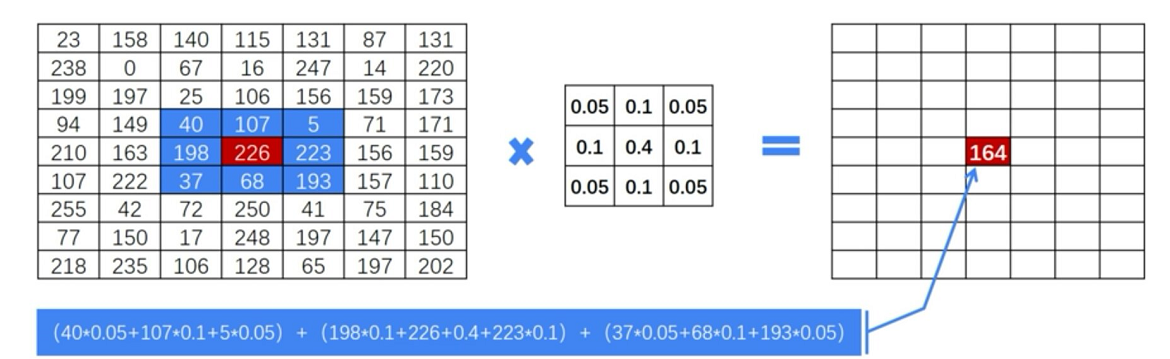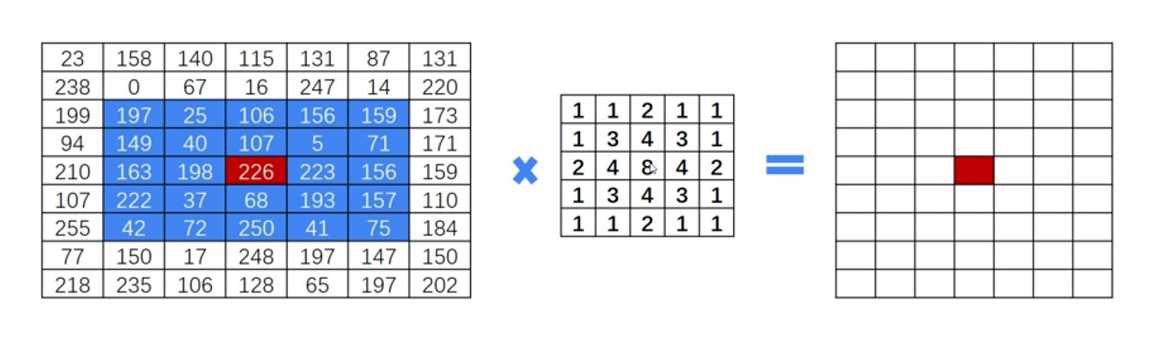Python中OpenCV主要调用GaussianBlur函数，如下：
dst = cv2.GaussianBlur(src, ksize, sigmaX)

$K(3,3)=\left[ \begin{matrix} 0.05 & 0.1 & 0.05 \\ 0.1 & 0.4 & 0.1 \\ 0.05 & 0.1 & 0.05 \end{matrix} \right] \tag{5}$
$K(5,5)=\left[ \begin{matrix} 1 & 1 & 2 & 1 & 1 \\ 1 & 3 & 4 & 3 & 1 \\ 2 & 4 & 8 & 4 & 2 \\ 1 & 3 & 4 & 3 & 1 \\ 1 & 1 & 2 & 1 & 1 \\ \end{matrix} \right] \tag{4}$

#encoding:utf-8
import cv2
import numpy as np
import matplotlib.pyplot as plt

#读取图片
source = cv2.cvtColor(img,cv2.COLOR_BGR2RGB)

#高斯滤波
result = cv2.GaussianBlur(source, (3,3), 0)

#显示图形
titles = ['Source Image', 'GaussianBlur Image']
images = [source, result]
for i in xrange(2):
plt.subplot(1,2,i+1), plt.imshow(images[i], 'gray')
plt.title(titles[i])
plt.xticks([]),plt.yticks([])
plt.show()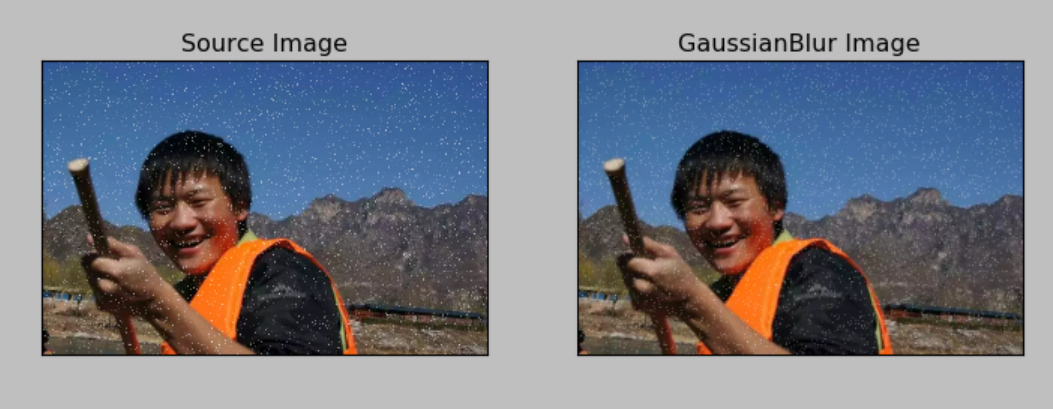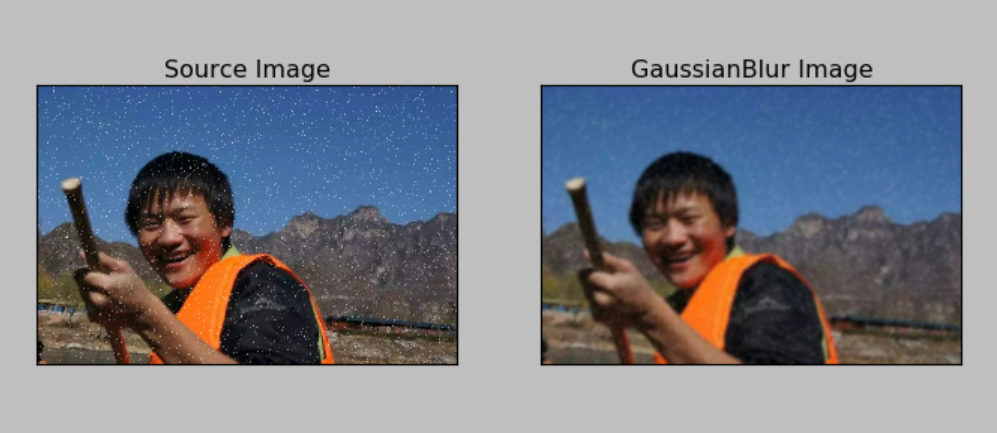# 五.中值滤波

1.概念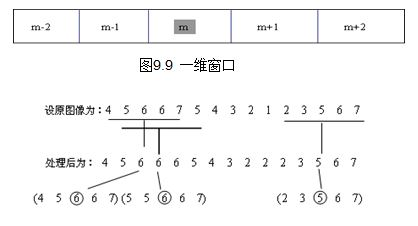2.代码
OpenCV主要调用medianBlur()函数实现中值滤波。图像平滑里中值滤波的效果最好。
dst = cv2.medianBlur(src, ksize)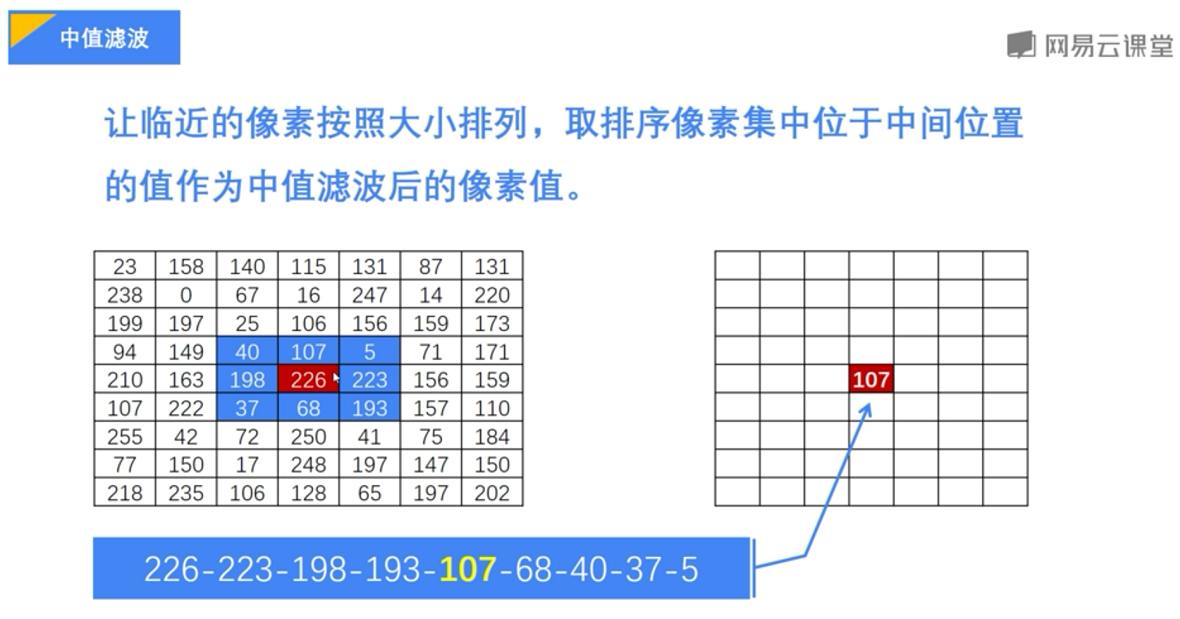#encoding:utf-8
import cv2
import numpy as np
import matplotlib.pyplot as plt

#读取图片

#高斯滤波
result = cv2.medianBlur(img, 3)

#显示图像
cv2.imshow("source img", img)
cv2.imshow("medianBlur", result)

#等待显示
cv2.waitKey(0)
cv2.destroyAllWindows()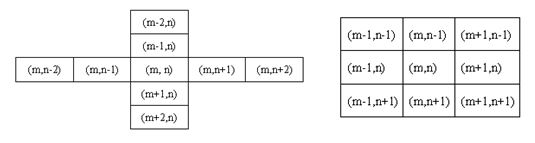（By：Eastmount 2018-09-01 早8点 https://blog.csdn.net/Eastmount/）

2013-06-10 17:01:54 insaneguy 阅读数 1195
• ###### 机器学习之概率与统计推断

本课程讲解机器学习算法所需概率和统计推断知识。概率部分包括概率公理及推论、条件概率、贝叶斯公式、随机变量及其概率函数（CDF／pdf）、常用概率分布及其均值、方差；统计推断部分包括大数定律和中心极限定理、极大似然估计、贝叶斯估计，估计的评价、偏差-方差平衡。课程还会讲解假设检验的基本概念。

20464 人正在学习 去看看 AI100讲师

	IplImage *pImgTmp = cvCloneImage(m_pImg);

// 第二种边界处理：对边界特殊处理
for (i = 0; i < m_pImg->width; i++)
{
for (j = 0; j < m_pImg->height; j++)
{
CvScalar color;
CvScalar colorTmp;
int m, n, cnt = 0;
color.val = color.val = color.val = 0;
for (m = i - nKernelSize/2; m <= i+nKernelSize/2; m++)
{
for (n = j-nKernelSize/2; n <= j+nKernelSize/2; n++)
{
// 处理边界和中心像素
if ( (m == i && n == j) || m < 0 || m >= m_pImg->width
|| n < 0 || n >= m_pImg->height )
continue;

cvGetPixel(pImgTmp, n, m, colorTmp);
color.val += colorTmp.val;
color.val += colorTmp.val;
color.val += colorTmp.val;
cnt++;
}
}
// 取邻域中像素的平均值
color.val /= cnt;
color.val /= cnt;
color.val /= cnt;
cvSetPixel(m_pImg, j, i, color);
}
}
cvReleaseImage(&pImgTmp);

void  cvGetPixel(const IplImage* pIplImg, int row, int col, CvScalar &color)
{
if (col >= pIplImg->width || col < 0 ||
row >= pIplImg->height || row < 0 )
return;

uchar *p = ((uchar*)(pIplImg->imageData + pIplImg->widthStep * row + col * 3));
color = cvScalar(p, p, p);
}

void  cvSetPixel(IplImage* pIplImg, int row, int col, CvScalar color)
{
if (col >= pIplImg->width || col < 0 ||
row >= pIplImg->height || row < 0 )
return;

uchar *p = ((uchar*)(pIplImg->imageData + pIplImg->widthStep * row + col * 3));
p = color.val;
p = color.val;
p = color.val;
}3×3模板结果：5×5模板结果：21×21模板结果：2020-03-16 09:29:03 Ibelievesunshine 阅读数 282
• ###### 机器学习之概率与统计推断

本课程讲解机器学习算法所需概率和统计推断知识。概率部分包括概率公理及推论、条件概率、贝叶斯公式、随机变量及其概率函数（CDF／pdf）、常用概率分布及其均值、方差；统计推断部分包括大数定律和中心极限定理、极大似然估计、贝叶斯估计，估计的评价、偏差-方差平衡。课程还会讲解假设检验的基本概念。

20464 人正在学习 去看看 AI100讲师

均值滤波，是图像处理中常用的手段，从频率域观点来看均值滤波是一种低通滤波器，高频信号将会去掉。均值滤波可以帮助消除图像尖锐噪声，实现图像平滑，模糊等功能。理想的均值滤波是用每个像素和它周围像素计算出来的平均值替换图像中每个像素。

以3*3均值滤波器为例，均值滤波器算法原理如下图：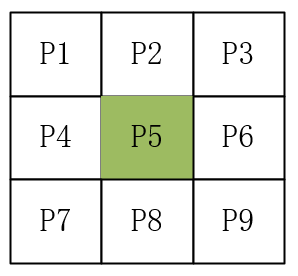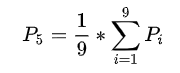↑

python 源码：

import cv2

import numpy as np

# mean filter

def mean_filter(img, K_size=3):

H, W, C = img.shape

out = np.zeros((H + pad * 2, W + pad * 2, C), dtype=np.float)

tmp = out.copy()

# filtering

for y in range(H):

for x in range(W):

for c in range(C):

out[pad + y, pad + x, c] = np.mean(tmp[y: y + K_size, x: x + K_size, c])

return out

# Mean Filter

out = mean_filter(img, K_size=5)

# Save result

cv2.imwrite("out.jpg", out)

cv2.imshow("result", out)

cv2.waitKey(0)

cv2.destroyAllWindows()原图  ↑模板大小5*5，均值滤波后的图像 ↑

可以看到，均值滤波后，图像中噪声虽然有所减弱，但是图像变模糊了。因为均值滤波器过滤掉了图像中的高频分量，所以图像的边缘都变模糊了。（去除一定量椒盐噪声，可以考虑使用中值滤波）

2019-09-16 20:12:07 weixin_44225182 阅读数 946
• ###### 机器学习之概率与统计推断

本课程讲解机器学习算法所需概率和统计推断知识。概率部分包括概率公理及推论、条件概率、贝叶斯公式、随机变量及其概率函数（CDF／pdf）、常用概率分布及其均值、方差；统计推断部分包括大数定律和中心极限定理、极大似然估计、贝叶斯估计，估计的评价、偏差-方差平衡。课程还会讲解假设检验的基本概念。

20464 人正在学习 去看看 AI100讲师

 t=imread('a1.jpg');
[m,n,z]=size(t);
t1=imnoise(t,'gaussian',0,0.02);
imshow(t1),title('加入高斯噪声后')
t2=t;
for i=1:m-2
for j=1:n-2
t2(i+1,j+1,:)=round(t2(i,j,:)/9+t2(i,j+1,:)/9+t2(i,j+2,:)/9+t2(i+1,j,:)/9+t2(i+1,j+1,:)/9+t2(i+1,j+2,:)/9+t2(i+2,j,:)/9+t2(i+2,j+1,:)/9+t2(i+2,j+2,:)/9);
end
end
figure,
subplot(1,2,1),imshow(t2),title('3*3均值滤波');
t3=t;
for i=1:m-4
for j=1:n-4
t3(i+2,j+2,:)=round(t3(i,j,:)/25+t3(i,j+1,:)/25+t3(i,j+2,:)/25+t3(i,j+3,:)/25+t3(i,j+4,:)/25+t3(i+1,j,:)/25+t3(i+1,j+1,:)/25+t3(i+1,j+2,:)/25+t3(i+1,j+3,:)/25+t3(i+1,j+4,:)/25+t3(i+2,j,:)/25+t3(i+2,j+1,:)/25+...
t3(i+2,j+2,:)/25+t3(i+2,j+3,:)/25+t3(i+2,j+4,:)/25+t3(i+3,j,:)/25+t3(i+3,j+1,:)/25+t3(i+3,j+2,:)/25+t3(i+3,j+3,:)/25+t3(i+3,j+4,:)/25+...
t3(i+4,j,:)/25+t3(i+4,j+1,:)/25+t3(i+4,j+2,:)/25+t3(i+4,j+3,:)/25+t3(i+4,j+4,:)/25);
end
end
subplot(1,2,2),imshow(t3),title('5*5均值滤波');
t1=imnoise(t,'salt & pepper',0.3);
figure,imshow(t1),title('加入椒盐噪声后')
t2=t;
for i=1:m-2
for j=1:n-2
t2(i+1,j+1,:)=round(t2(i,j,:)/9+t2(i,j+1,:)/9+t2(i,j+2,:)/9+t2(i+1,j,:)/9+t2(i+1,j+1,:)/9+t2(i+1,j+2,:)/9+t2(i+2,j,:)/9+t2(i+2,j+1,:)/9+t2(i+2,j+2,:)/9);
end
end
figure,
subplot(1,2,1),imshow(t2),title('3*3均值滤波');
t3=t;
for i=1:m-4
for j=1:n-4
t3(i+2,j+2,:)=round(t3(i,j,:)/25+t3(i,j+1,:)/25+t3(i,j+2,:)/25+t3(i,j+3,:)/25+t3(i,j+4,:)/25+t3(i+1,j,:)/25+t3(i+1,j+1,:)/25+t3(i+1,j+2,:)/25+t3(i+1,j+3,:)/25+t3(i+1,j+4,:)/25+t3(i+2,j,:)/25+t3(i+2,j+1,:)/25+...
t3(i+2,j+2,:)/25+t3(i+2,j+3,:)/25+t3(i+2,j+4,:)/25+t3(i+3,j,:)/25+t3(i+3,j+1,:)/25+t3(i+3,j+2,:)/25+t3(i+3,j+3,:)/25+t3(i+3,j+4,:)/25+...
t3(i+4,j,:)/25+t3(i+4,j+1,:)/25+t3(i+4,j+2,:)/25+t3(i+4,j+3,:)/25+t3(i+4,j+4,:)/25);
end
end
subplot(1,2,2),imshow(t3),title('5*5均值滤波');